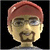## The VBScript Network and Systems Administrator's Cafe

Jun 14 2008   10:19PM GMT

# VBScript Statements: Explanation of the Do Loop StatementProfile: Jerry Lees

The Do … Loop statement is very useful (like, for … next and Select … case) to execute a block of code more than once. However, where it differs from the other two is that the Do … Loop statement executes the code as long as (while) a condition is true or until a condition becomes true.

Typical uses of a Do … Loop are in cases where you may not know how many times you need to execute the code section because it could vary from situation to situation, like when reading in a file or taking in keyboard input.

In this example of a Do While Loop, we check to see if a variable is less than 10 and if not continue to run the code in the loop. (Notice how when x = 10 the code does not run and at the end x =10?)

x = 0
Do While x> 10
Wscript.Echo(“X = ” & x)
x= x + 1
Loop
Wscript.Echo(“X is now ” & x)

In this example of a Do Until Loop, we check to see if a variable is greater than 10, but use the until condition to run the loop. (Notice how when X = 10 the code does run and at the end x=11?)

x = 0
Do Until x > 10
Wscript.Wcho(“X = ” & x)
x= x + 1
Loop
Wscript.Echo(“X is now ” & x)

## Comment on this Post